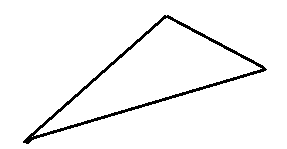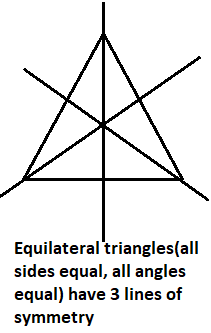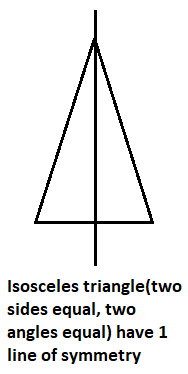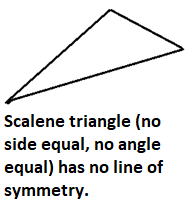QUESTION

# How many lines of symmetry does the following given figure (Scalene triangle) have ?A. 3B. 2C. 1D. 0

Hint: We had to only use the definition of line of symmetry for any shape which says that if any figure has x number of lines of symmetry then there will be x possible lines which divide that figure into two equal parts (i.e. mirror images). And then check the number of lines in the scalene triangle which divide it into two mirror images.